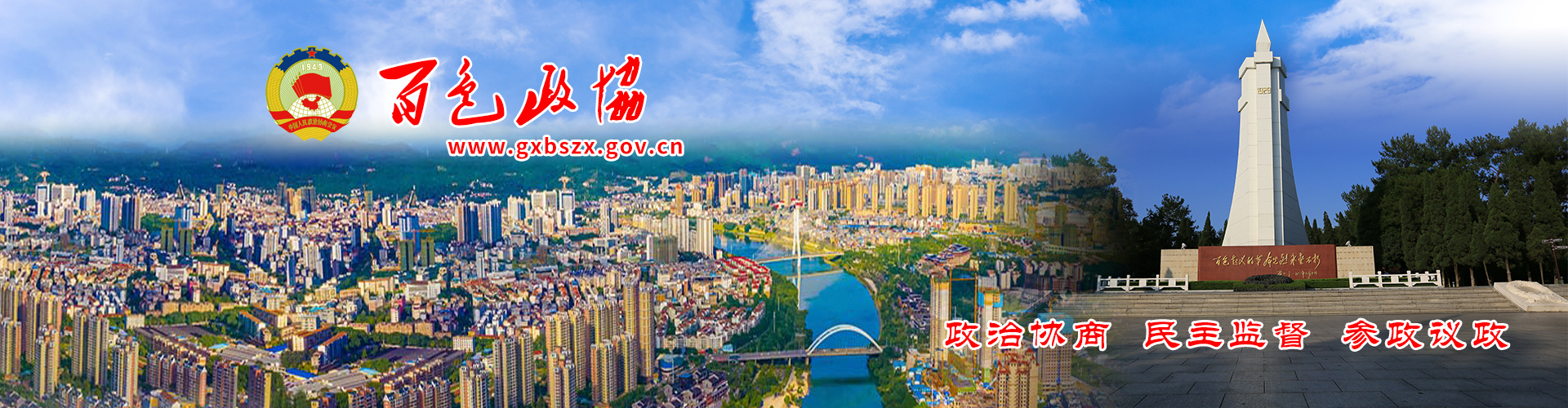# 政协第五届百色市委员会委员名单

2022年01月10日 20时46分   阅读：184098 次   来源：广西百色政协网   作者：   责任编辑：杨煜智 【  +放大   -缩小  】

(按界别以姓氏笔画为序)

中国共产党

马 方(女，壮族)  韦 刚  韦荣黎(壮族)  韦 胜(壮族)  左吉兵      石国怀(苗族)

李志光(瑶族)  李维民(壮族)  岑万兴(壮族)  陆政时(壮族)

陈世邦  林 健(壮族)  罗胜明(瑶族)  黄志琳(女，壮族)   黄克胜(壮族)

黄建宁  梁韶玫(女，壮族)   蒋正波  韩 旭(壮族)  覃 平(壮族)

蒙冬莉 (女，瑶族)    黎仕兴(壮族)

民革、民盟、民建、民进、农工党、致公党、九三学社

乃振峰(壮族)  王 慧(女)  王大理(壮族)  文衍红  邓耀德  龙昌文  刘 涛

农 锷(壮族)  李 鑫(壮族)  陆月然(壮族)  陆国语(壮族)  林剑勇(瑶族)

周永尧(壮族)  姚 明(壮族)  姚源果  莫洪军(壮族)  黄 伟  黄永全(壮族)

黄承忠(壮族)  黄金贵(壮族)   黄彩玲(女，壮族)  梁显瓜(女，壮族)   鄢永库(壮族)

廖英良(女)  丁丽华(女，壮族)  卢媛英(女，瑶族)   叶国云  闭晟毓(壮族)

农有宁(壮族)  余剑冰  邹高丽(女)  陈晓慧(女，壮族)   林志强(壮族)

罗胜益(壮族)  黄伟旗(壮族)  黄 慧(女，壮族)  黄毅勤(女)  梁晓碧(女，壮族)

梁清梅(女)  覃炳伟 (壮族)  谭晓领(女，壮族)

共青团

韦彩玉(女，瑶族)  刘牡丹(女，瑶族)   杨胜军(苗族)  张红梅(女)  林锦涛

欧 伟(壮族)  周旭存(壮族)    陵丽清(女，壮族)  黄仕攀(彝族)

黄祝青(女，侗族)   黄振诚(壮族)  梁 晗 (壮族)  隆盛营(壮族)  覃晓荷(女，壮族)

廖志雄(壮族)

总工会

丁立华  王林林(白族)  韦昌涛(壮族)    李顺超  肖 兵

陆 泉(女，壮族) 陆晓燕(女，壮族) 陈德阜(壮族)  莫树亮(壮族)  黄世全  黄 欣(壮族)

黄波(壮族)  黄学梅(女，壮族)       黄祖亮   黄海波(壮族)  梁梓盛(壮族)  蒋立民

妇女联合会

邓迎春(女，瑶族)   甘政虹(女)   苏宇梅(女，仡佬族)  李 英(女，壮族)   杨轶晖(女)

陆丹萍(女，壮族)  罗新苗(女，壮族)   胡淑薇(女)   凌 滢(女，壮族)

郭金香(女，仡佬族)   龚艳清(女，壮族)   彭 慧(女)  覃 燕(女，壮族)

曾凤春(女，壮族)

工商业联合会

韦才平(壮族)  田仁流  田桂云(女，壮族)  邢俊杰  汤存龙

苏秀东(女，壮族)   李 宁  杨万生    吴江华  应 映(女)  沈朝卫

陈逢凯(女)  林木金  林宝峰    周 南  胡鸿雁  班俊安(壮族)

顾家奕  唐寸舒(女，壮族)  黄广振  黄彩雪(女，壮族)  梁 平

梁绍邱(壮族)   梁富谋(壮族)  梁 筱(女，壮族)   覃国洪(壮族)   蒙培养(壮族)

虞静云(女，苗族)   潘隆春

科学技术协会

马燕春(女，苗族)   邓雅娜(女，壮族)   李秀青(女，苗族)  杨再芬  吴友明(苗族)

张启胜(壮族)  张征宏(壮族)  罗俊书(壮族)       周  纲（瑶族）  周连芳(女，壮族)

骆映箐(女，壮族)   黄同屋(壮族)  黄宏飞(壮族)

归国华侨联合会

韦剑强(壮族)  卢玥琳(女，壮族)  吕子慧(女)  刘仰齐  许秀芬(女，壮族)

林 格(壮族)         邹秉城（壮族）  罗伟胜(壮族)  徐卫良  黄玲珍(女，壮族)

梁家迅(壮族)  曾 妮(女)  温海燕(女，壮族)

文学艺术

王 迅  阮清龙       李雄涛  杨 阳(女，苗族)  岑长青(壮族)   林 雄(壮族)

周 会(壮族)   莫维铭(壮族)  徐少华  唐毓飞(壮族)  陶丽群(女，壮族)

黄肖婷(女，壮族)  黄胜章(壮族)  黄福龙(壮族)  覃 琪(女，壮族)

科学技术

马成明(仡佬族)  王天跃  王 伟(壮族)   王忠海  石秀芳(女，壮族)

农诚海(壮族)  杨 艳(女)  吴荫松(壮族)  陆永成(壮族)  罗显华

黄志辉  黄武军(壮族)  黄莉雯(女，壮族)   黄辉成(壮族)

社会科学

卢炳宋(瑶族)   阮 鸣(女，壮族)   李 萍(女，壮族)  苏其军  杨富莲(女)

张耀平(女，壮族)  骆心云(苗族)   黄 明(壮族)  黄秋琴(女，壮族)

黄慧翔(女，壮族)   廖  晖(壮族)

经济

韦 鸣(壮族)  韦学智(壮族)  石 英(壮族)  卢 梁  冯文伟  汤晓军(壮族)

农温校(壮族)  苏芳芳(女，壮族)   杨正乾  旷 松  张 华  张 金(壮族)

张金显  张葵香(女)  陆国建(壮族)  陆 海(壮族)  陈 颖(女)  罗秋瑛(女，壮族)

赵丽珍(女，瑶族)   赵映杰(女，壮族)  唐星善  黄贵华(壮族)  曾 云(壮族)

蒙汉良(壮族)  蒙应祥(壮族)  蔡文姬(女，壮族)

农业农村

马天祥(壮族)  韦 妮(女，壮族)   韦祖乾(壮族)  朱 贺  伍奕蓉(女)  刘映驿(女)

苏  民(壮族)  李婉静(女)  杨自爽  吴德广  岑 山(壮族)       张向磊  陈国民

林群英(女，壮族)     赵小强(壮族)  姚俞任(女)

黄 宁(壮族)  黄若伦(壮族)    黄海珠(女)  黄 鑫(壮族)  傅旭安

教育

韦忠兵(壮族)  韦勇忧(女，壮族)   刘序畅  许凤盼(女，壮族)  李飞雪(女，壮族)

李和平(壮族)  李荣善(壮族)  杨 敏(壮族)  陈大治  陈诗明

林  莲(女，壮族)   屈迟文  黄凤萍(女，壮族)   黄明锦(壮族)  黄建平(壮族)

黄  雷(壮族)  黄增向(女，壮族)   蒙 龙(壮族)  谭欣欣(女，壮族)   黎 明(壮族)

文新体广电

王正庭(壮族)  韦丽莎(女，壮族)  韦照民(壮族)  农长城 (壮族)  李春玲(女)

杨 敏(女，壮族)  张素兰(女，壮族)   罗 丽(女，壮族)     凌英甲(壮族)

黄 凤(女，仡佬族)   黄尚礼(壮族)  黄国俊(壮族)  黄海珍(女，壮族)  黄斯杰(壮族)

黄 靖  黄毅贞(女，壮族)  梁开平(壮族)  梁明英(女，壮族)   廖长安(壮族)

黎 炜(壮族)  戴 强

医药卫生

韦凤兰(女，瑶族)  李鸿翔(壮族)  吴 勇  岑 俊(壮族)  何 璇(女，壮族)

辛荣根(壮族)  张孟新  陈培静  赵素勤(女，壮族)  黄龙贵(壮族)  黄军科(壮族)

黄丽明(女，壮族)  黄明矿(壮族)  黄诗航

黄 毅(壮族)  梁 晓(女，壮族)

民族宗教

韦晓东(壮族)  闭鸿飞(壮族)  许国章(壮族)  农海燕(女，壮族)   李果果(女，瑶族)

李荣表(壮族)  杨明治(苗族)  杨 翊(女，苗族)   何连师(女，瑶族)

陈玉莲(女，瑶族)   陈国宇(苗族)   周雨玲(女，壮族)  钟小燕(女，瑶族)

班丽芳(女，壮族)   袁 劭  黄子锋(彝族)  盘文波

特邀人士(党政军警等)

邓志辉  黄金亮(壮族)  黄李慧(壮族)  纪海峰  李常政(壮族)  李 杰(壮族)

李林洋(瑶族)   李永孔(壮族)  梁绍宏(壮族) 龙国芳  马俊杰(仡佬族)  宁程鹏(壮族)

潘仲文(壮族)   彭绍东  韦 啸(壮族)  肖洪林  张晓晔(女)

特邀人士(港澳人士)

李元君  李劲源  李健民  吴卓璟  何文峰  何美娥(女)   余柏盈  陈英棋

陈 宜  陈彩玉(女)   林志坚  林冠东  林满珍(女)   罗翰荣  郑俊瀚  赵文炎

洪金乐  黄云键  曾耀祖  谢天泽  蔡子乐